## Monday, September 14, 2020

### Cover points with tiles

In  a problem is posted:

Given $$n$$ points and a collection of tiles (rectangles) of given size, try to cover all all points with the minimum number of tiles.

There are a number of things to consider:

• Are the tiles of equal size or have they different sizes?
• Can tiles overlap?
• After minimizing the number of tiles, should we minimize the total size of the selected tiles, so we select the smaller tiles when possible.

#### Data

Let's generate some data, assuming tiles have different sizes:

----     19 PARAMETER p  location of points

x           y

p1       17.175      84.327
p2       55.038      30.114
p3       29.221      22.405
p4       34.983      85.627
p5        6.711      50.021
p6       99.812      57.873
p7       99.113      76.225
p8       13.069      63.972
p9       15.952      25.008
p10      66.893      43.536
p11      35.970      35.144
p12      13.149      15.010
p13      58.911      83.089
p14      23.082      66.573
p15      77.586      30.366
p16      11.049      50.238
p17      16.017      87.246
p18      26.511      28.581
p19      59.396      72.272
p20      62.825      46.380
p21      41.331      11.770
p22      31.421       4.655
p23      33.855      18.210
p24      64.573      56.075
p25      76.996      29.781
p26      66.111      75.582
p27      62.745      28.386
p28       8.642      10.251
p29      64.125      54.531
p30       3.152      79.236

----     19 PARAMETER r  size of rectangles

x           y

r1       12.183      15.270
r2       25.769      32.506
r3       15.344      11.024
r4       27.554      28.637
r5       21.681      20.761
r6       17.291      17.393
r7       13.915      38.003
r8       21.398      33.502
r9       19.001      13.764
r10      32.466      12.077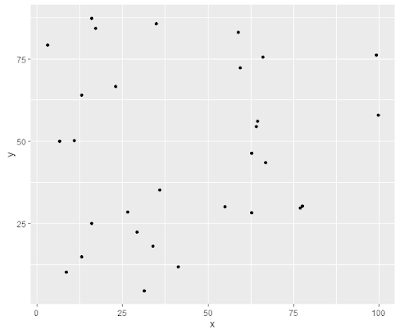Random points

#### Model

To model this problem, we introduce binary variables $$\mathit{select}_j \in \{0,1\}$$: $\mathit{select}_j = \begin{cases} 1 & \text{if tile j is selected}\\ 0 & \text{otherwise}\end{cases}$ and a continuous variable $$\mathit{place}_{j,c}$$ indicating the $$x$$ and $$y$$ coordinates of (selected) tile $$j$$. To be precise: it is the left-lower corner of the rectangle. The set $$c$$ is simply $$c=\{x,y\}$$. In addition we need a variable indicating if a point is covered by a tile:$\mathit{cov}_{i,j}=\begin{cases} 1 & \text{if point i is covered by tile j} \\ 0 &\text{otherwise}\end{cases}$

The model can look like:

MIP Model
\begin{align}\min &\sum_j \color{darkred}{\mathit{select}}_j \\ & \color{darkred}{\mathit{place}}_{j,c} \le \color{darkblue}p_{i,c}+\color{darkblue}M\cdot(1-\color{darkred}{\mathit{cov}}_{i,j}) && \forall i,j,c \\ &\color{darkred}{\mathit{place}}_{j,c}+\color{darkblue}r_{j,c}\ge\color{darkblue}p_{i,c}-\color{darkblue}M\cdot(1-\color{darkred}{\mathit{cov}}_{i,j}) && \forall i,j,c \\ & \color{darkred}{\mathit{cov}}_{i,j} \le \color{darkred}{\mathit{select}}_j && \forall i,j \\ & \sum_j \color{darkred}{\mathit{cov}}_{i,j} \ge 1 && \forall i \\ & \color{darkred}{\mathit{select}}_j \in \{0,1\} \\ & \color{darkred}{\mathit{cov}}_{i,j} \in \{0,1\} \end{align}

Our points are drawn from $p_{i,c}\sim U(0,100)$ So it make sense to restrict the placement of tiles to: $0 \le \mathit{place}_{j,c} \le 100-r_{j,c}$ Using this, a good value for $$M$$ is $$M=100$$.

#### Results

----     55 VARIABLE z.L                   =        7.000  objective

----     55 PARAMETER tile  placement of tiles

x           y           w           h

r1       53.928      67.819      12.183      15.270
r2        5.652                  25.769      32.506
r4       27.484       6.507      27.554      28.637
r5       78.131      55.464      21.681      20.761
r6        5.791      49.181      17.291      17.393
r8       56.188      22.573      21.398      33.502
r10       2.517      75.169      32.466      12.077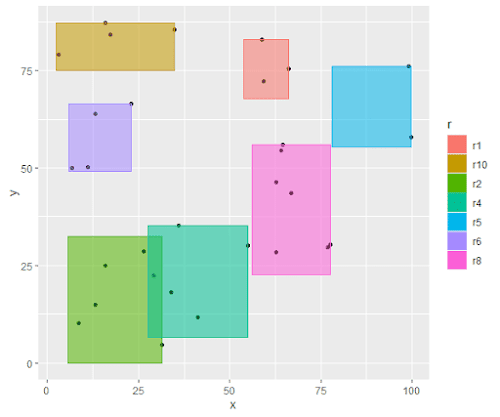Points are covered by tiles

#### No overlap

We can add no-overlap constraints  to the model soo that no pair of tiles can overlap. Just to be sure, I'll allow tiles to go outside the area $$[0,100]\times [0,100]$$. Of course, that also has an impact on the value for $$M$$. The new solution looks like: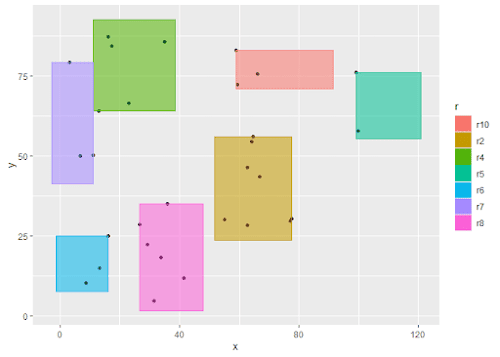With no-overlap constraints

#### Same-sized tiles

If all tiles are identical, we just have a special case of our original problem. It may make sense to add an ordering constraint: $\mathit{select}_j \le \mathit{select}_{j-1}$ We can also consider additional symmetry-breaking constraints, such as $\mathit{place}_{j,x} \ge \mathit{place}_{j,x-1}$ It requires some experimentation if these constraints pay off in performance.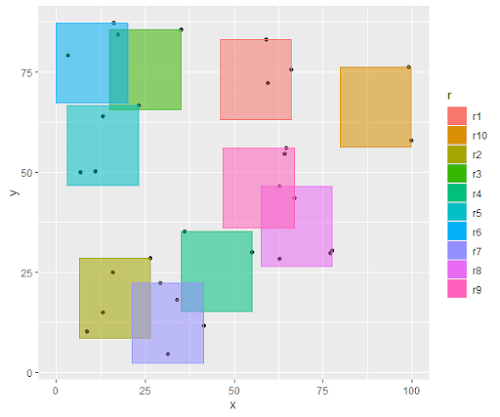All tiles have the same size

In this example all the times are $$(20 \times 20)$$. Below is a larger example with $$n=50$$ points and $$(10 \times 10)$$ tiles.Larger example

#### Set-covering formulation for equal-sized tiles

If the problem has all equal-sized tiles, we can employ a very different algorithm.  For this we need to generate candidate locations in advance.  One way to generate those is as follows:

1. $$k:=0$$
2. For each point with coordinates $$(x_1,y_1)$$:
1. For each point with coordinates $$(x_2,y_2)$$ such that $$x_1 \le x_2 \le x_1+w$$ and $$y_1-h\le y_2\le y_1+h$$:
1. Form the candidate rectangle $$(x_1,y_2)-(x_1+w,y_2+h)$$
2. $$k := k+1$$
3. Store as $$\mathit{loc}_{k,c}=(x_1,y_2)$$
4. Find all points $$i$$ inside the candidate rectangle, and store this inside a mapping $$\mathit{contain}_{k,i}$$
Now we can solve the set-covering problem :

Set-covering Model
\begin{align}\min & \sum_j \color{darkred}{\mathit{select}}_j \\ & \sum_j \color{darkblue}{\mathit{contain}}_{j,i} \cdot \color{darkred}{\mathit{select}}_j \ge 1 && \forall i \\ & \color{darkred}{\mathit{select}}_j \in \{0,1\}\end{align}

where $\mathit{contain}_{j,i} = \begin{cases} 1 & \text{if candidate rectangle j contains point i}\\ 0 & \text{otherwise}\end{cases}$

This model solves very fast.

#### A multi-criteria version

Instead of just minimizing the number of tiles, we can subsequently minimize the total area covered by the tiles. I.e. use smaller tiles if possible. This is essentially a multi-objective problem. We used here a lexicographic approach (i.e. do two solves in sequence). In our experiment, we reduced the total size of the selected tiles from 3672.5809 to 3004.0812.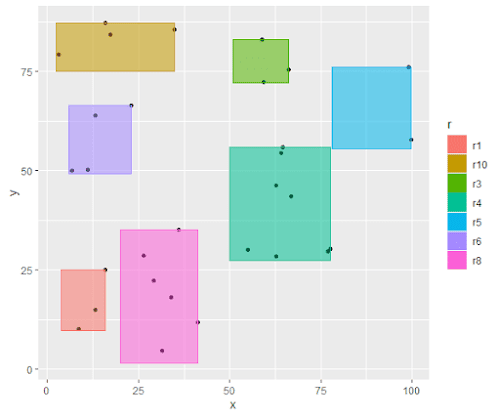Multi-objective model results

Obviously, this additional step makes only sense if the tiles have different sizes.

#### GAMS Models

As requested, here are some of the source files.

Model for tiles with different sizes.

 set   i 'points to cover'  /p1*p30/   j 'rectangles' /r1*r10/   c 'coordinates' /x,y/ ; parameter    A 'side of area' / 100 /    M 'big-M'    p(i,c) 'location of points'    r(j,c) 'size of rectangles' ; p(i,c) = uniform(0,A); r(j,c) = uniform(10,40); M = A; display p,r; binary variable select(j)  'select tile'; positive variable place(j,c) 'placement of tile'; place.up(j,c) = A-r(j,c); variable z 'objective'; binary variable cov(i,j); equations    obj    cover1    cover2    cover3    cover4 ; obj..  z =e= sum(j, select(j)); cover1(i,j,c)..  place(j,c) =l= p(i,c)+M*(1-cov(i,j)); cover2(i,j,c)..  place(j,c)+r(j,c) =g= p(i,c)-M*(1-cov(i,j)); cover3(i,j)..  cov(i,j) =l= select(j); cover4(i).. sum(j,cov(i,j)) =g= 1; model m1 /all/; option optcr=0, threads=8; solve m1 minimizing z using mip; select.l(j) = round(select.l(j)); parameter tile(j,*) 'placement of tiles'; tile(j,c) = place.l(j,c)*select.l(j); tile(j,'w')$select.l(j) = r(j,'x'); tile(j,'h')$select.l(j) =  r(j,'y'); display z.l,tile;

Set-covering model for equal-sized tiles.

 set   i 'points to cover'  /p1*p50/   j 'candidate rectangles' /r1*r1000/   contain(j,i) 'points contained in rectangle j'   c 'coordinates' /x,y/ ; parameter    A 'side of area' / 100 /    p(i,c) 'location of points'    r(c) 'size of rectangles'  /x 10, y 10/    loc(j,c)  'locations of candidate rectangles' ; p(i,c) = uniform(0,A); display p,r; *------------------------------------------------------------ * generate candidate rectangle locations *------------------------------------------------------------ alias (i,ii,iii); scalar k / 0 /; loop(i,    loop(ii$(p(ii,'x') >= p(i,'x') and p(ii,'x') <= p(i,'x')+r('x') and abs(p(ii,'y')-p(i,'y')) <= r('y')), k = k + 1; loop(j$(ord(j)=k),          loc(j,'x') = p(i,'x');          loc(j,'y') = p(ii,'y')-r('y');          contain(j,iii) = p(iii,'x') >= loc(j,'x') and p(iii,'x') <= loc(j,'x')+r('x') and                           p(iii,'y') >= loc(j,'y') and p(iii,'y') <= loc(j,'y')+r('y')       );    ); ); *------------------------------------------------------------ * set covering model *------------------------------------------------------------ binary variable select(j)  'select tile'; variable z 'objective'; equations    obj    cover(i) ; obj..  z =e= sum(j, select(j)); cover(i)..  sum(contain(j,i),select(j)) =g= 1; model m1 /all/; option optcr=0; solve m1 minimizing z using mip; *------------------------------------------------------------ * reporting *------------------------------------------------------------ parameter tile(j,*) 'placement of tiles'; tile(j,c) = loc(j,c)*select.l(j); tile(j,'w')$select.l(j) = r('x'); tile(j,'h')$select.l(j) =  r('y'); display z.l,tile;
The R code to plot the results looks like:

library(ggplot2)

p1       17.175      84.327
p2       55.038      30.114
p3       29.221      22.405
p4       34.983      85.627
p5        6.711      50.021
p6       99.812      57.873
p7       99.113      76.225
p8       13.069      63.972
p9       15.952      25.008
p10      66.893      43.536
p11      35.970      35.144
p12      13.149      15.010
p13      58.911      83.089
p14      23.082      66.573
p15      77.586      30.366
p16      11.049      50.238
p17      16.017      87.246
p18      26.511      28.581
p19      59.396      72.272
p20      62.825      46.380
p21      41.331      11.770
p22      31.421       4.655
p23      33.855      18.210
p24      64.573      56.075
p25      76.996      29.781
p26      66.111      75.582
p27      62.745      28.386
p28       8.642      10.251
p29      64.125      54.531
p30       3.152      79.236

r <- read.table(text="r x y w h
r1       53.928      67.819      12.183      15.270
r2       15.562       2.638      25.769      32.506
r4       50.032      27.438      27.554      28.637
r5        7.540       4.247      21.681      20.761
r6        5.791      49.181      17.291      17.393
r7       85.897      38.222      13.915      38.003
r10       2.517      75.169      32.466      12.077

ggplot(pt, aes(x=x , y=y)) + geom_point() +
geom_rect(data=r, aes(xmin=x,xmax=x+w,ymin=y,ymax=y+h,color=r,fill=r,alpha=0.1))+
guides(alpha=FALSE)


#### Conclusion

The small MIP model presented above can be used as a basis for alternative, related problems. For equal-sized tiles, a faster set-covering model is shown.